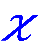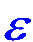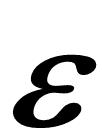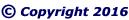"CLICK" below image; or, above blue ellipse to navigate.
 Emergent Ellipsoid Geometry Symbolic Dark Matter (Pulsoid)Bisect the Center Line with a Rotation Line. The Elliptical Constant (EC), epsilon,, equals One.
 The Salient Structural Parts (SSP) of an Emergent Ellipse (EE) are all integers when the Pulse (P) is an integer.
 As an extension of Line FG, draw Line AB, which is the Major Diameter of both the ellipse through points A, B, D, and J; and, the ellipse through points A, B, E, and K. Line AF equals Lines BG, CH, and CI.
 With Points H and I as Foci, draw an ellipse through Point E. Point K is on the ellipse, which is referred to as a Vector ellipse. All Vector Lines, such as Lines DF and EI, are equal.
 With Points F and G as Foci, draw an ellipse through Point D. Point J is on the ellipse, which is referred to as a radius ellipse.
 Extend the Center Line as required Draw a Vector Line EI that is equal to Line GH from Point I to the Center Line. Draw a Vector Line DF that is equal to Line FI from Point F to the Center Line.
 Transpose Pythagorean Triangles FGJ and HIK as indicated. Extend the Center Line as required.
 Inscribe Circles (in-circles) FGJ and HIK with centers atand respective diameters LM and NO. Bisect Lines FG and HI with Center Lines.
 Construct a paired Pythagorean Triangle FGJ in accordance with base, side, and  hypotenuse as indicated in the below Table of Formulas. A pair of Pythagorean Triangles have short sides, radius (r) and base (b), which vary by the Elliptical Constant (EC) =.
 Construct a Pythagorean Triangle HIK in accordance with base, side, and  hypotenuse as indicated in the below Table of Formulas. Pythagorean Triangles are triangles with a right angle; and, all sides that are integers.

 Pulsoid Theorem (PTm) V =P2= Elliptical Constant (EC) = One
 x = Integer (base 10)=b/2
 All Vector Lines, all Pulse Lines,  and all soliton Lines are equal. .
 Table of Formulas
 DF & EI = Vector (V)=(b/2)2 = x2
 AF & CI = Pulse (P)=b/2 = x
 Pythagorean Triangles
 FGJ = Pythagorean radius Triangle
 CF = soliton (s)=(b2–2b)/4 = x2–x
 FG = wave (w)=b2/2–b = 2(x2–x)
 GJ = radius (r)=b–= 2x–FJ = hypotenuse (h)=b2/2–b+= 2x2–2x+HIK = Pythagorean Vector Triangle
 HC = V-soliton (Vs)=b/2 = x
 HI = base (b), V-wave, (Vw) = 2x
 HK = V-radius (Vr)=b2/4 –= x2–IK = V-hypotenuse (Vh)=b2/4+= x2+Resoloids (in-circles)
 FGJ = radius-Resoloid (in-circle) = rR
 LM = r-diameter (rd)=b–2= 2(x–)L = r-radius (rr)=b/2–= x–HIK = Vector-Resoloid (in-circle) = VR
 NO = VR-diameter (VRd)=b–2= 2(x–)N = VR-radius (VRr)=b/2–= x–The Pythagorean Theorem, a2 + b2 = c2, is an an incredible coincidence of number theory, which arises from the Emergent Ellipsoid and the Natural source of Mathematics.
 A Pythagorean Triangle Pair (PTP) can be mapped to every integer greater than One. Four angles (two of the six angles are right angles) and all six sides of a Pythagorean Triangle Pair are of unlike values.
 The simplest equation for the in-diameter of a circle inscribed within any right triangle is:
 An in-diameter of a right triangle equals side "a" plus side "b" minus the hypotenuse "c".
 Amazingly, the diameters of both circles inscribed within each different Pythagorean Triangle, of a given pair, are even integers,  that are . . . identical to one another. Thus, the radii of the inscribed circles are also equal integers.
More amazingly,
for every Emergent Ellipsoid (EEd),
regardless of size or shape,
the inscribed circle radii
are equal to the
Pulse (P) minus the Elliptical Constant,.

It can be said that PTP phenomena
is at the root of many of the enigmas of
mathematics and physics . . . and of
all else that exists.

 "CLICK" top image; or, below blue ellipse to navigate.One must continuously ask: Why? Why? Why?; and, Why? again. And, realize that Fundamental Nature is the source of all Mathematics!
 Summary Epsilon equals One Proof of One Inverse Square Law Elliptical Constant Duality of Infinity
 Natural Function Brunardot Theorem revised Fibonacci Sequence Challenge to Academe Pulsoid Theorem Fundamental Intrinsic Time
 Salient Structural Parts Universal Locus Antimatter Heaven/God/Hell Philogic Entanglement

--- Page 9 ---